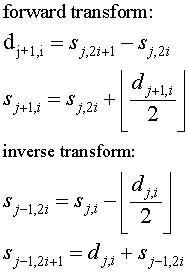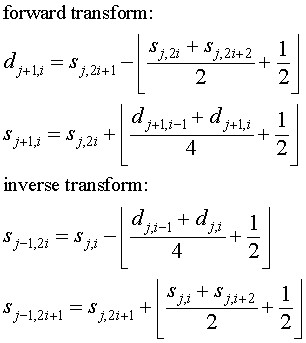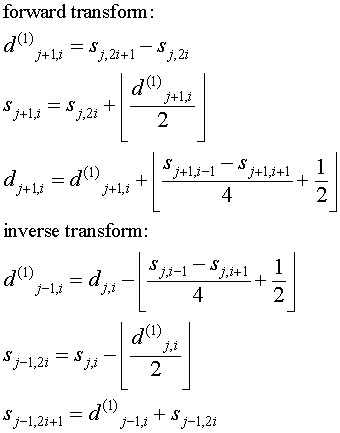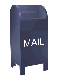## Lossless Wavelet Compression

### Introduction

This web page discusses lossless data compression using integer to integer wavelet transforms and an integer version of the wavelet packet transform.

The lossless compression discussed here involves 1-D data. However, these algorithms directly generalize to 2-D data (e.g., images) . Applications for lossless compression include compression of experimental data and compression of financial time series (for example, the bid/ask time series that arise from intra-day trading).

Usually a technique like integer to integer wavelet compression is applied to a large data set (like an image) as the first step in the compression process. A second data coding step is applied. Ideally the first step will result in repeated value (either sequential repeats or multiple occurrences of a given value), so additional compression can be achieved by a coding algorithm like Huffman or run length coding.

The motivation behind this web page is not data compression per se. Wavelet compression is a form of predictive compression. Predictive compression algorithms can be used to estimate the amount of noise in the data set, relative to the predictive function. Turning this around, wavelet compression, especially wavelet packet compression can be used to estimate the amount of determinism in a data set. This can be useful in time series forecasting, since a region with low noise and high determinism may be a region that is more predictable. In terms of wavelet compression, a region that is relatively compressible may be more predictable. This web page lays the foundation for the related web page Wavelet compression, determinism and time series forecasting. The example time series used here are stock market close prices for a small set of large capitalization stocks.

Along with this discussion on lossless wavelet compression, I have published the C++ source code for these algorithms. format).

### Data Compression

Data that is compressed via a lossless compression algorithm can exactly recovered by the decompression algorithm. Lossless compression algorithms are used to compress data where loss cannot be tolerated. For example, text, experimental data or compiled object code. The lossless compression program gzip (GNU Zip), a Free Software compression program, is widely used on UNIX and GNU/Linux systems for lossless compression.

Lossy compression algorithms are not perfectly invertible. The decompressed result of a lossy compression algorithm is an approximation of the orignal data. Lossy compression algorithms are frequently used to compress images and digitized sound files. Computer display and sound playback devices have limited resolution. The human eye and ear are also limited in their resolution. In many cases selected image and sound data can be removed without reducing the perceived quality of the result.

One notable exception to lossy image compression is medical images. While selected data can be removed from these images without observable loss of quality, no one wants to take the chance of removing detail that may be important. Medical images are frequently transmitted using lossless compression.

This web page and the algorithms published here concentrate on 1-D financial time series data (e.g., stock market close price). These compression algorithms will generalize directly to 2-D data. In many 2-D image compression algorithms, the first step of the compression algorithm converts the 2-D image data into a linear vector. For example, a 1,024 x 1,024 pixel image can be converted to linear form by linearizing the rows of the image to create a vector of 1,048,576 pixel elements (see  for an excellent discussion of image linearization).

The data sets used as examples here are small relative to image and sound files. A wavelet compression algorithm applied to a large data file would be followed by a coding compression step, like Huffman or run length coding. Such a coding step is of little use for a small data set, since the result of the compression algorithm frequently has few repeat values.

### Data Compression and Determinism

Data compression is a fascinating topic when considered by itself. This web page examines lossless data compression as a method to estimate the amount of determinism, relative to noise, in a financial time series. Estimating the amount of determinism (or noise content) in a signal region may be useful in time series forecasting. This is discussed on the related Web page Wavelet compression, determinism and time series forecasting.

Deterministic data is generated by some underlying process which is not entirely random. For example, the water level of the Nile river is influenced by the seasonal rains, which provide an underlying deterministic process. The water level on any given day is influenced by daily rain, which is a random event. This creates a time series for the Nile river water level that consists of deterministic information mixed with random information, or noise.

If a magic compression algorithm existed that could exactly model all deterministic processes, the data left after these deterministic values were subtracted would be noise (data generated by a non-deterministic process). Given a starting set of values, our magic compression function would be able to predict all the deterministic values in the data set. So given the value s0 the magic compression function could calculate all of the other values s1 to sn. If there was no noise in the deterministic data the magic compression algorithm could compress the data set to a small set of values, consisting of the initial starting state and the length of the data set to be generated. At the other end of the spectrum, is a data set this is composed entirely of values generated by a non-deterministic process (e.g., noise). This data set cannot be compressed to any significant degree.

Cellular automata, fractal functions, the calculation of pi to N places and pseudo-random number generators are examples of functions that can generate extremely complex results from a simple starting point. There is no magic compression function that will discover the function and starting value for given a data sequence. In practice, a compression algorithm uses, at most, a small set of approximation functions. This function is used to "predict" values in the data set from earlier values. For example, we might chose a linear compression function that predicts that the value si+1 will be equal to si. The difference between this prediction function and the data set is the incompressible information. This is the Lifting Scheme version of the Haar wavelet transform. Another compression function might be chosen which predicts that the point si lies on a line running between the points si-1 and si+1. The difference between the predicted value at si and the actual value at si is the incompressible data. This is the linear interpolation wavelet. Other prediction functions include 4-point polynomial interpolation and spline interpolation.

In wavelet terminology, the predictive function is referred to as the wavelet function. The wavelet function is paired with a scaling function. The wavelet function acts as a high pass filter. The scaling function acts as a low pass filter, which avoids aliasing (large jumps in data values).

Most modern compression techniques use a two step process:

1. A predictive compression function (perhaps a wavelet transform) is applied in the first step. If the predictive compression function is a good fit for the data set, the result will be a new data set with smaller values, and more repetition.

2. A coding compression step that attempts to represent the data set in its minimal form. A coding compression algorithm like Huffman coding is applied to the result of the predictive step. Ideally the predictive step will result in smaller values, with some values that are repeated.

Predictive compression yields good results for image compression, where the change from pixel to pixel is not an entirely random process (assuming that the image is of something other than random noise). A predictive step would be useless for text compression however, since there is no underlying deterministic process in a natural language text stream that can be approximated with a function. Text compression relies on data representation compression (coding compression).

### A Digression on Shannon Entropy

If we have a compression program, it would be nice if there was a metric that would tell us how efficient our compression program is. The Shannon entropy equation is frequently referenced in the compression literature. What can Shannon entropy equation tell us?

### Integer to Integer Wavelet Transforms

The wavelet transform takes an input data set and maps it to a transformed data set. When a floating point wavelet transform is applied to an integer data set, the transform maps the integer data into a real data set. For example, an integer data set S is shown below.

S = {32, 10, 20, 38, 37, 28, 38, 34, 18, 24, 18, 9, 23, 24, 28, 34}

When the Lifting Scheme version of the Haar transform is applied to the data set, the integer data set is mapped to the set of real numbers shown below.

```25.9375
-7.375
9.25 10.0
8.0 3.5 -7.5 7.5
-22.0 18.0 -9.0 -4.0 6.0 -9.0 1.0 6.0
```

If the input data set is not random and a wavelet function is chosen that can approximate the data on one or more scales, the wavelet transform will produce a result that represents the original data set as a set of small values, with a few large values that are of the magnitude of the original data. This is a round about way of stating that the original data set has been compressed using a predictive compression algorithm.

The object of a compression algorithm is to represent the original data in fewer bits. If the wavelet transform maps an integer data set into a floating point data set, no compression may have been achieved, since the fractional digits must be represented. In fact, a set of floating point results may require more bits than were needed to represent the original integer data set.

If the compression algorithm is lossy, the result of the wavelet transform can be rounded to integer values or small values may be set to zero. By modifying the result of the wavelet transform, perfect invertibility is lost and the original input data can not be exactly regenerated. A lossy compression algorithm might be appropriate for the image compression used for Web page images, but it would be totally unacceptable for compressing the data from a chemistry experiment or a financial time series. Lossy compression also does not provide as good an estimate for calculating the amount of deterministic information in a time series, since some information has been discarded.

Integer to integer wavelet transforms map an integer data set into another integer data set. These transform are perfectly invertible and yield exactly the original data set. An excellent discussion of integer to integer wavelet transforms can be found in , particularly sections 3 and 4. This web page relies heavily on this paper (of course any mistakes are mine).

Any wavelet transform can be generalized to an integer to integer wavelet transform. For example in  an integer to integer version of the Daubechies D4 wavelet transform is presented.

I have implemented three integer to integer Lifting Scheme wavelet transforms. This are listed below, along with the equations for the forward and inverse transform. One of the elegant things about the wavelet Lifting Scheme is that the inverse transform is the mirror of the forward transform, with the + and - operators interchanged.

1. An integer version of the Lifting Scheme version of the Haar transform. In image processing this is known as the S transform.2. An integer version of the linear interpolation wavelet transform. The linear interpolation transform "predicts" that an odd element will be on a line between its two even neighbors. The difference between this "prediction" and the actual value becomes the wavelet coefficient (sometimes called the detail coefficient). The scaling function is simply the average of the original even and odd elements.3. An integer version of the Cohen-Daubechies-Feauveau (3,1) wavelet transform. This is sometimes referred to as the TS transform in the image processing literature.The wavelet lifting scheme is discussed on the related web page Basic Lifting Scheme Wavelets. The equations above correspond to lifting scheme steps.

The notation used here is based on the notation in , so I'll briefly try to explain it.

The forward wavelet transform takes N elements in step j and calculates N/2 detail coefficients (shown as d above) and N/2 scaled values (shown as s above). The scaled values become the input for the next step of the wavelet transform (which is why these values are shown with the subscript j+1). In the next step, Nj+1 = Nj/2.

The inverse wavelet transform in step j rebuilds the sj-1,i and sj-1,i+1 (even and odd elements) from the dj and sj values.

### Compression and Small Data Sets

Compression is usually applied to large data sets. The estimation of the power of the compression algorithm is based on the number of bits needed to represent the compressed data set.

Compared to media data, like images, sound or video, the amount of data in a time series that we are trying to develop a forecast (for example, these "close" price for a stock) is relatively small. The window of data that the compression algorithm is applied to in forecasting may be even smaller.

A coding step (for example, Huffman coding or the Variable Bit Length Coding proposed in ) applied to the result of the predictive step attempts to represent the data in a minimal number of bits. The coding step can find a more compact representation for the data when there are long runs of values (say 28) that can be represented in the same number of bits or when the frequency distribution of values is not uniform.

The wavelet result for a relatively small data set (say 64 or 128 values) will tend to have few repeat values (e.g., a frequency close to 1/N for each value, in the case of N values). Huffman and other "dictionary" based coding schemes work poorly in this case. Since the data set is relatively small, there may not be large runs of values that can be represented in the same number of bits.

The motivation for the work discussed here is to use compression (the number of bits needed to represent the data set after the transform) to estimate the amount determinism in the data set. If a high degree of compression is achieved then the wavelet algorithm closely approximated the original data set, leaving only small residual values. This indicates that there was a high degree of determinism, relative to the wavelet function.

Rather than applying a classic compression coding scheme to estimate the number of bits needed to represent the wavelet result, a simple total for the number of bits is calculated. The total number of bits needed for to represent the wavelet result is the sum of the bit widths for each element. For a real compression algorithm, where the result must be decompressed as well, this represents an impractical lower bound. In a real compression algorithm a sub-sequence of values would be allocated in a common number of bits per value. A header for the subsequence, perhaps with a count and a width indicator would proceed the sequence. These headers and the common value widths in a sequence increase the size of the compression result.

### Results

This section discusses the results of applying wavelet algorithms to a set of stock close price time series. In all cases these time series consist of 512 elements. The data was down-loaded from Yahoo. This data is in "decimalized" form (e.g., there are only two fractional decimal digits, representing US cents). The fixed point data is multiplied by 100 to convert it to integer form. The list of stocks is shown below, in Table 1.

Table 1

NYSE/NASDQ Symbol Company
aa Alcoa Aluminium
amat Applied Materials
ba Boeing
cof Capital One
ge General Electric
ibm IBM Corp.
intc Intel
mmm 3M Corp
mrk Merck
wmt Wal-Mart

The three integer to integer wavelet algorithms discussed above (Haar, linear interpolation and the TS transform) were applied to the data sets. An integer version of the wavelet packet transform, using the linear interpolation wavelet, was also used. The cost function for the wavelet packet transform was bit width.

To provide a comparison for the more complex wavelet algorithms, a simple difference compression algorithm was also applied (I refer to this as "delta"). A data set of N elements is replaced by the differences. In this simple algorithm

```  si = si - si-1.
```

For an array indexed from 0, i = 1 to N-1. The element at s0 is the reference element, which is unchanged. The next element at s1 is replaced by the difference between that element and s0.

The "delta" algorithm is very simple and has a time complexity of N, where as the wavelet algorithm is Nlog2N.

Table 2 shows the results of the compression algorithms. The values are the total number of bits needed to represent the data set. This total is an impractical lower bound arrived at by adding up the number of bits needed to represent each element. While this lower bound is not practical for a real compression algorithm, it does provide a reasonable metric for comparing the results.

Table 2, Compression for 512 element data sets

Symbol Uncompressed delta Haar line TS wavelet packet (line)
aa 7188 4071 4291 4155 4134 3876
amat 7059 4199 4415 4214 4272 3966
ba 7572 4279 4467 4320 4302 4118
cof 7664 4603 4824 4571 4696 4368
ge 7423 4146 4341 4155 4210 3977
ibm 8140 4829 5013 4826 4842 4627
intc 7211 4355 4555 4379 4400 4115
mmm 8188 4592 4807 4613 4688 4479
mrk 7728 4315 4512 4273 4319 4144
wmt 7680 4285 4474 4213 4276 4030

The data for this table was generated by the compresstest.cpp code in the lossless/src subdirectory in the source code directory tree.

### Discussion

One surprising result is that the simple "delta" compression algorithm compares very well to the slower, more complicated wavelet algorithms, in terms of compression. In fact, in several cases the "delta" algorithm yields better results. Another interesting result is that in all cases the integer version of the wavelet packet algorithm yields the best result.

The wavelet packet algorithm yields a "best basis" set, where sections of the data set are represented by wavelet functions at different scales. The scale chosen is the scale that minimizes the cost function (in this case bit width). Another way to view this is that the wavelet packet function provides the best approximation for the data, relative to a given wavelet function.

The wavelet packet algorithm is also the slowest algorithm. Like all wavelet algorithms, it's time complexity is 0Nlog(N). However, the O factor will be bigger for the wavelet packet algorithm because of its more complicated data structure. The wavelet packet data structure must also be traversed by the cost function and the best basis function, which selects the best basis set.

The code needed to implement the wavelet packet algorithm is also larger, so it has a higher Kolmogorov complexity than either the simple "delta" algorithm or a standard wavelet algorithm.

Although the wavelet packet function is not without its extra cost, it is a better choice for estimating the determinism in a time series, since it provides a close fit for the data. In short term time series forecasting the wavelet packet algorithm would be applied to relatively small windows of data. The computational cost of the integer to integer wavelet packet algorithm is not so high that it could not be used in real time on a modern high speed processor.

### C++ Source Code and Documentation

The integer to integer wavelet transforms and the integer version of the wavelet packet transform reuses some of the software implemented for floating point wavelet transforms and the floating point wavelet packet algorithm. As a result, this software is included in the lossless subdirectory in source code for wavelet packets. A data subdirectory includes the test data sets (historical stock close prices down-loaded from Yahoo). The data subdirectory also include the yahooTS class which reads these data sets from disk.

The C++ source code for wavelet packets and lossless wavelet compression algorithms can be down-loaded from this web page.

The doxygen generated documentation can be found here

### References (Books)

• A Guide to Data Compression Methods by David Solomon, Springer Verlag, 2002

• Ripples in Mathematics by Jensen and la Cour-Harbo, Springer Verlag, 2001

• An Introduction to Kolmogorov Complexity and Its Applications by Ming Li and Paul Vitanyi, Second Edition, Springer Verlag, 1997

This book has been a pleasant surprise. The book is carefully written and understandable with a basic background in discrete math. The authors take great care to explain the notation they use and they write clearly. The book is dense with ideas (and mathematics), which makes it a time consuming read. I have a theory that fully understanding a book like this may take the reader as long as it took the authors to write the book.

### References Archived in the CiteSeer Research Index

1. State of the Art Lossless Image Compression Algorithms by S. Sahni, B.C. Vemuri, F. Chen, C. Kapoor, C. Leonard, and J. Fitzsimmons, October 30, 1997

2. Wavelet Transforms that Map Integers to Integers by A.R. Calderbank, Ingrid Daugbechies, Wim Sweldens, and Boon-Lock Yeo, 1996

Ian Kaplan
August 2002
Revised: